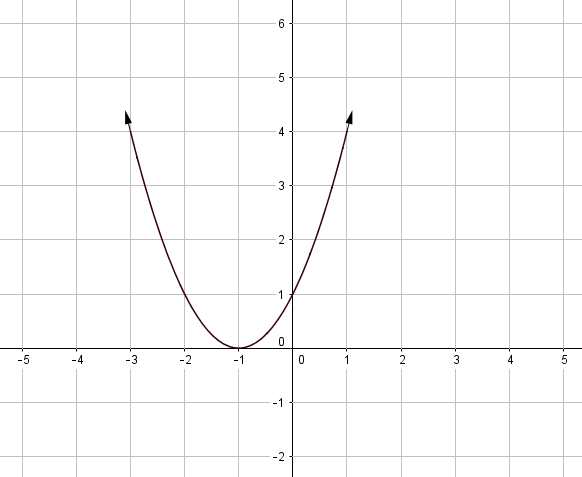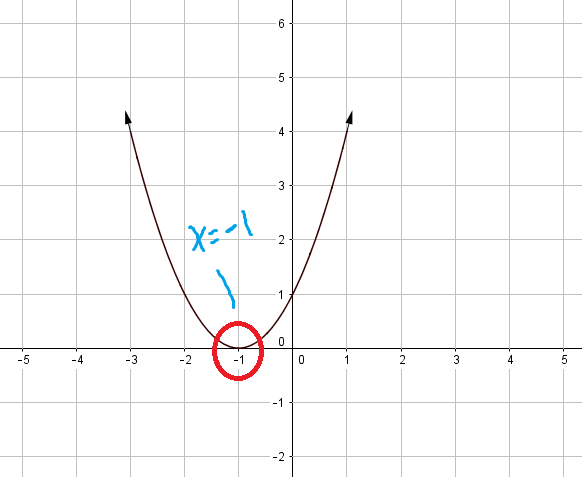﻿ Finding the zeros of a polynomial from a graph - MathBootCamps

# Finding the zeros of a polynomial from a graph

The zeros of a polynomial are the solutions to the equation p(x) = 0, where p(x) represents the polynomial. If we graph this polynomial as y = p(x), then you can see that these are the values of x where y = 0. In other words, they are the x-intercepts of the graph.

The zeros of a polynomial can be found by finding where the graph of the polynomial crosses or touches the x-axis.

Let’s try this out with an example!

## Example

Consider a polynomial f(x), which is graphed below. What are the zeros of this polynomial?To answer this question, you want to find the x-intercepts. To find these, look for where the graph passes through the x-axis (the horizontal axis).This shows that the zeros of the polynomial are: x = –4, 0, 3, and 7.

While here, all the zeros were represented by the graph actually crossing through the x-axis, this will not always be the case. Consider the following example to see how that may work.

## Example

Find the zeros of the polynomial graphed below.As before, we are looking for x-intercepts. But, these are any values where y = 0, and so it is possible that the graph just touches the x-axis at an x-intercept. That’s the case here!From here we can see that the function has exactly one zero: x = –1.

## Connection to factors

You may remember that solving an equation like f(x) = (x – 5)(x + 1) = 0 would result in the answers x = 5 and x = –1. This is an algebraic way to find the zeros of the function f(x). Each of the zeros correspond with a factor: x = 5 corresponds to the factor (x – 5) and x = –1 corresponds to the factor (x + 1).

So if we go back to the very first example polynomial, the zeros were: x = –4, 0, 3, 7. This tells us that we have the following factors:

(x + 4), x, (x – 3), (x –7)

However, without more analysis, we can’t say much more than that. For example, both of the following functions would have these factors:

f(x) = 2x(x+4)(x–3)(x–7)

and

g(x) = x(x+4)(x–3)(x–7)

In the second example, the only zero was x = –1. So, just from the zeros, we know that (x + 1) is a factor. If you have studied a lot of algebra, you recognize that the graph is a parabola and that it has the form$a(x+1)^2$, where a > 0. But only knowing the zero wouldn’t give you that information*.

*you can actually tell from the graph AND the zero though. Since the graph doesn’t cross through the x-axis (only touches it), you can determine that the power on the factor is even. But, this is a little beyond what we are trying to learn in this guide!## Subscribe to our Newsletter!

We are always posting new free lessons and adding more study guides, calculator guides, and problem packs.

Sign up to get occasional emails (once every couple or three weeks) letting you know what's new!